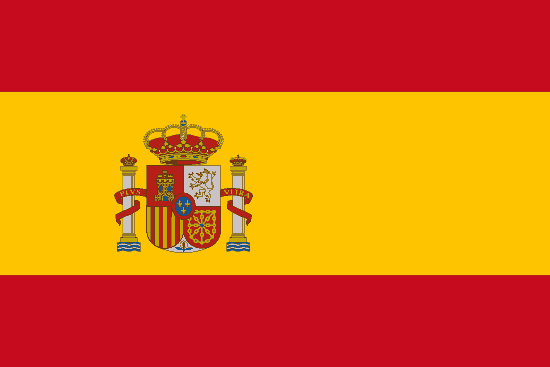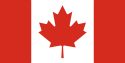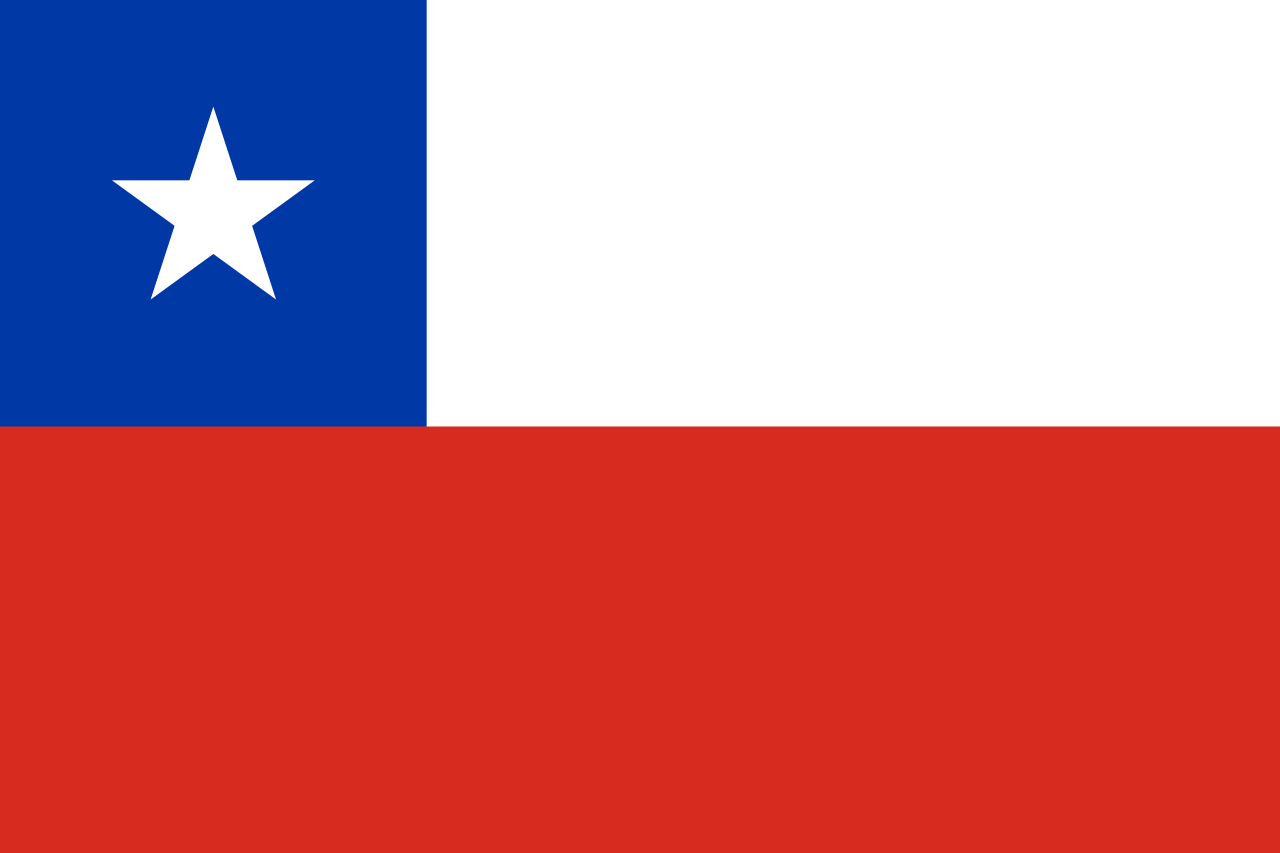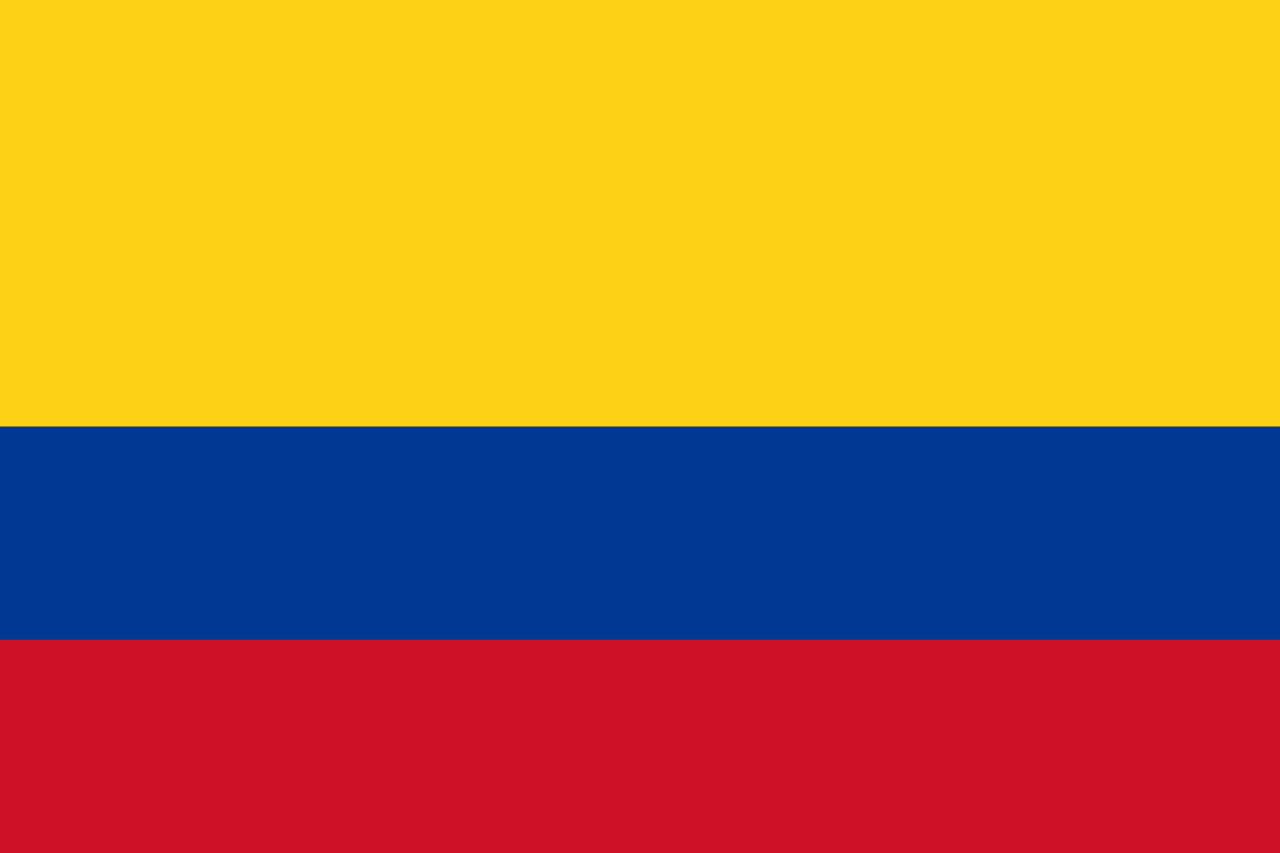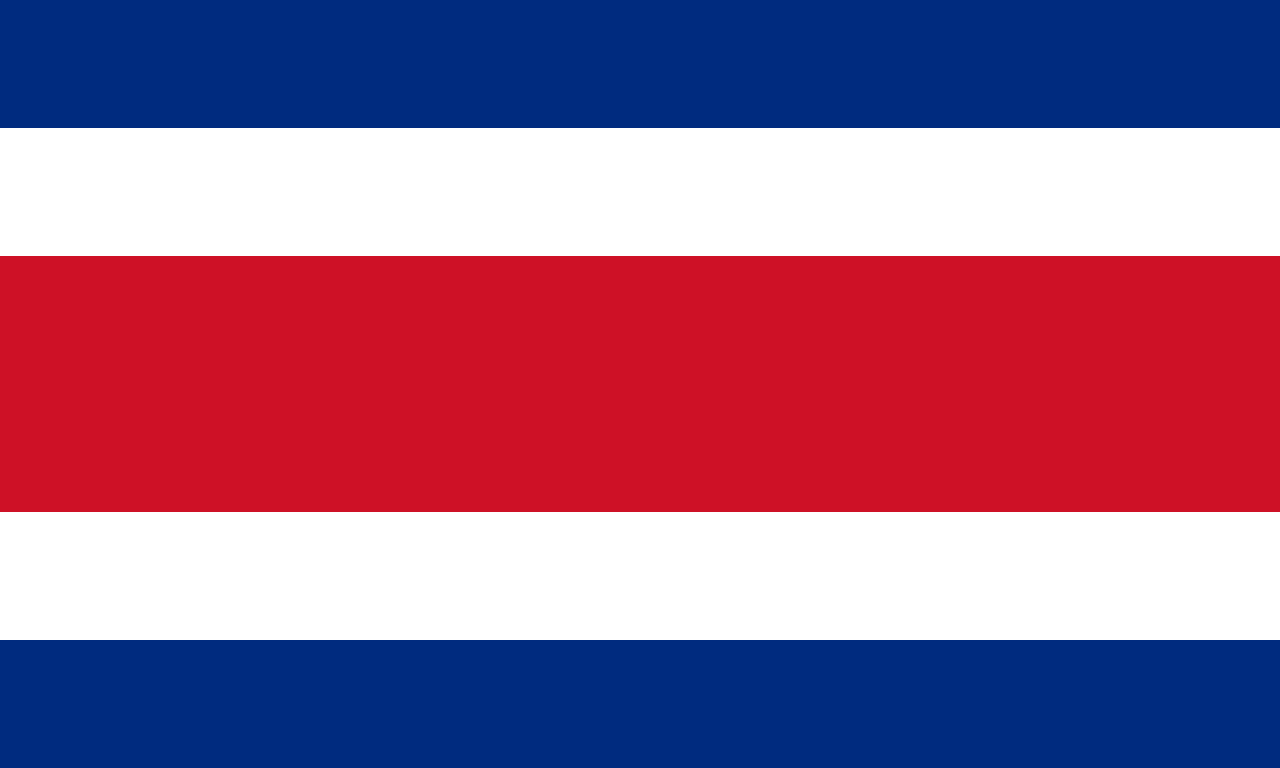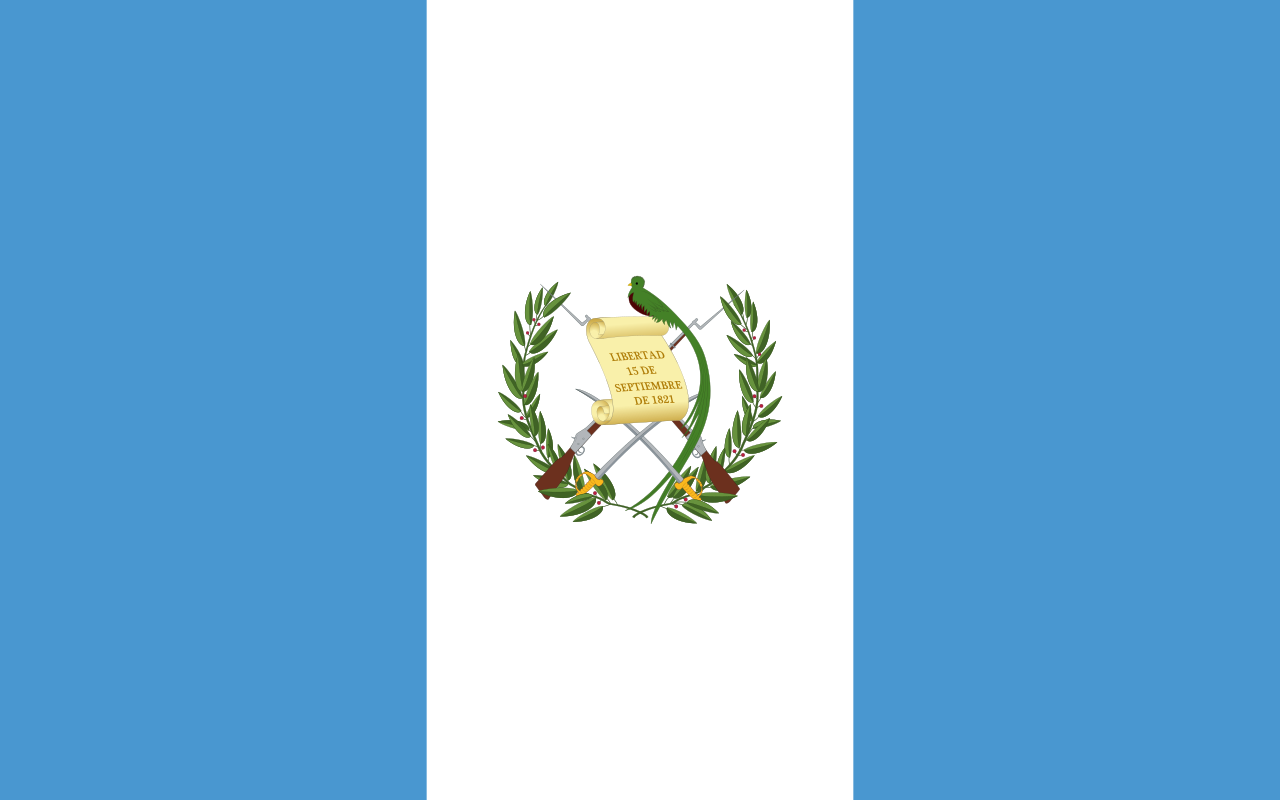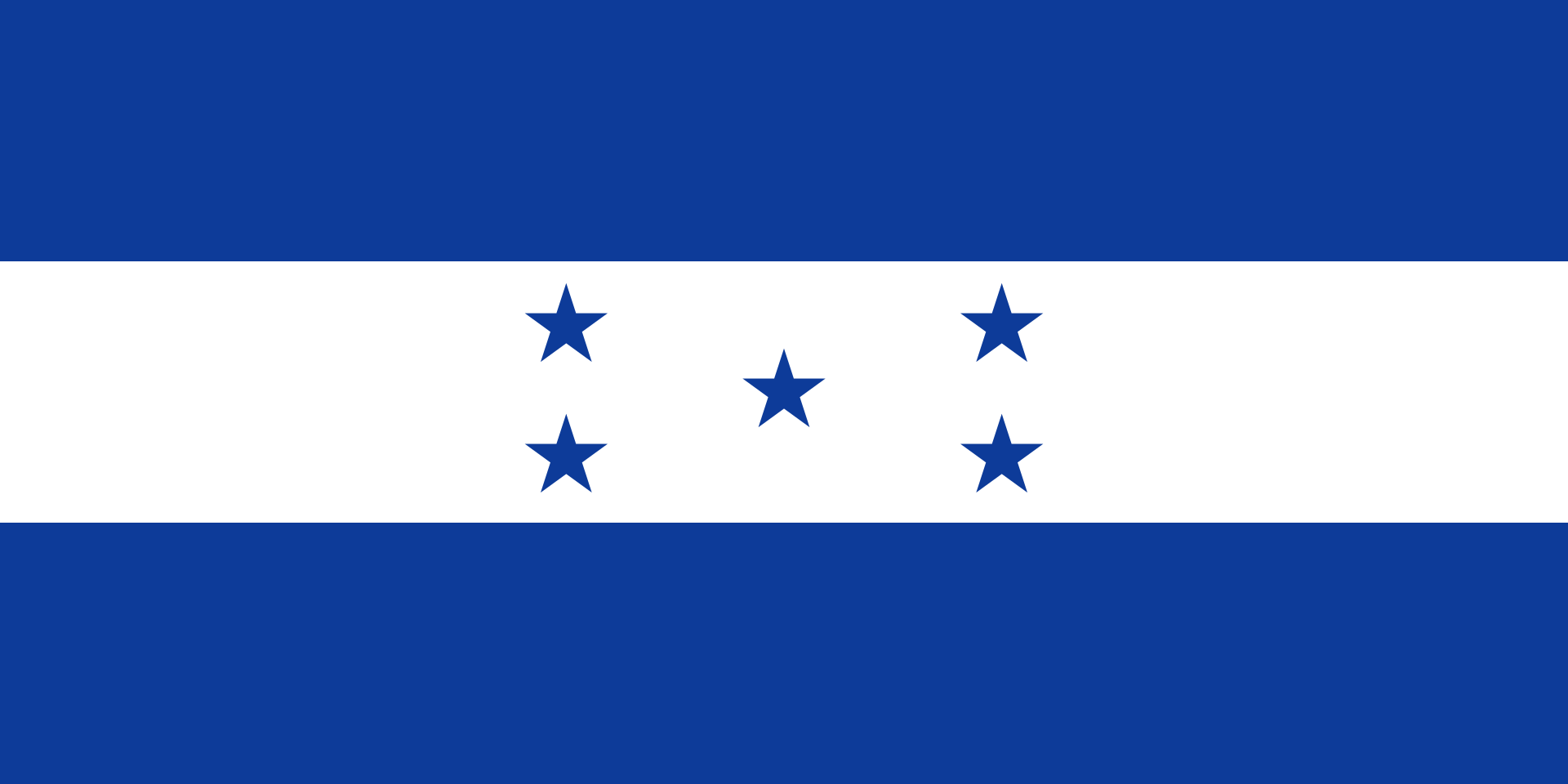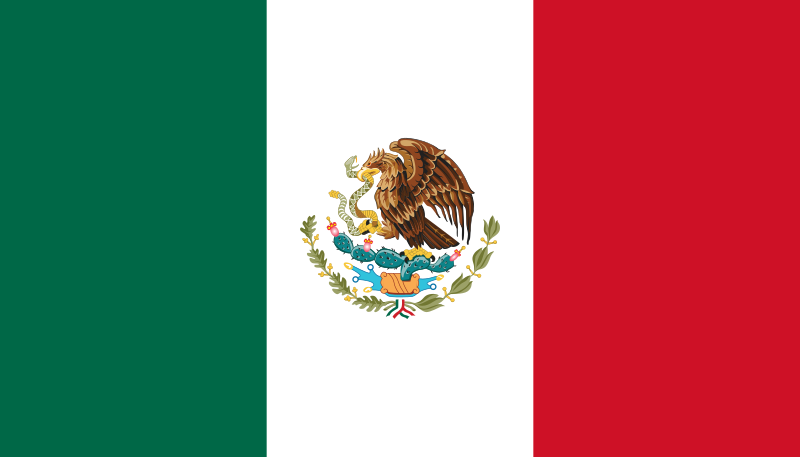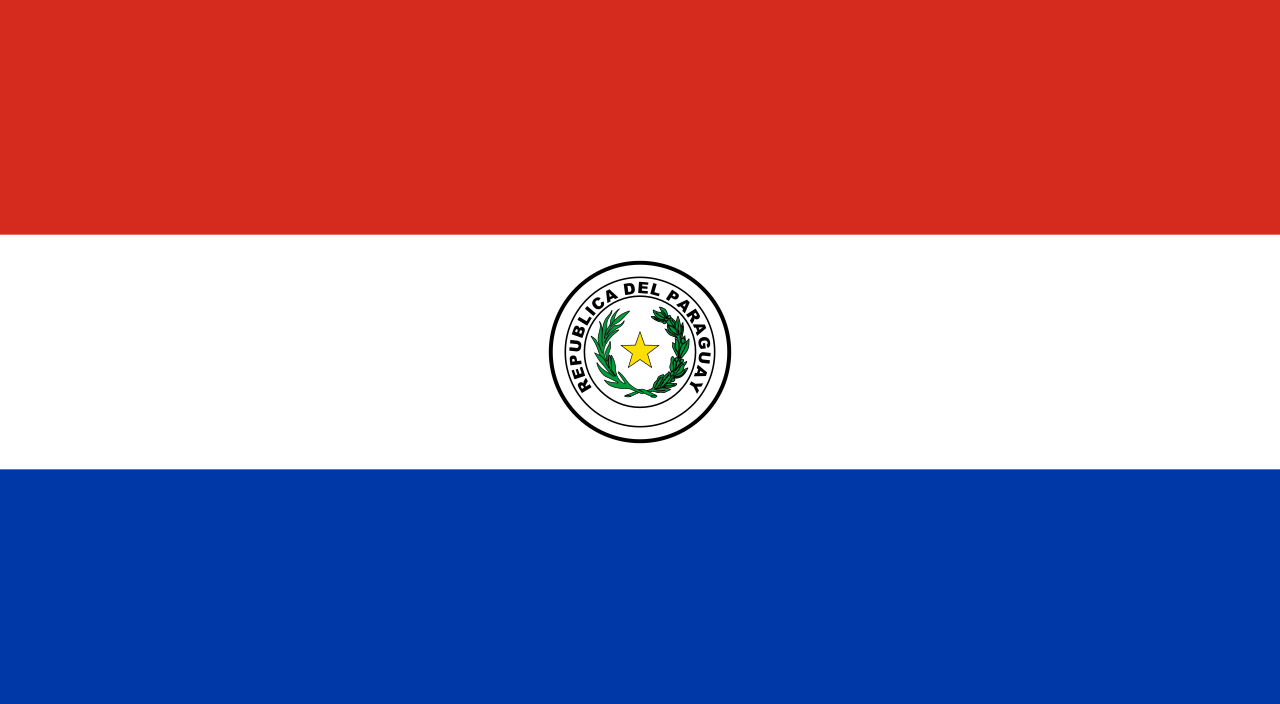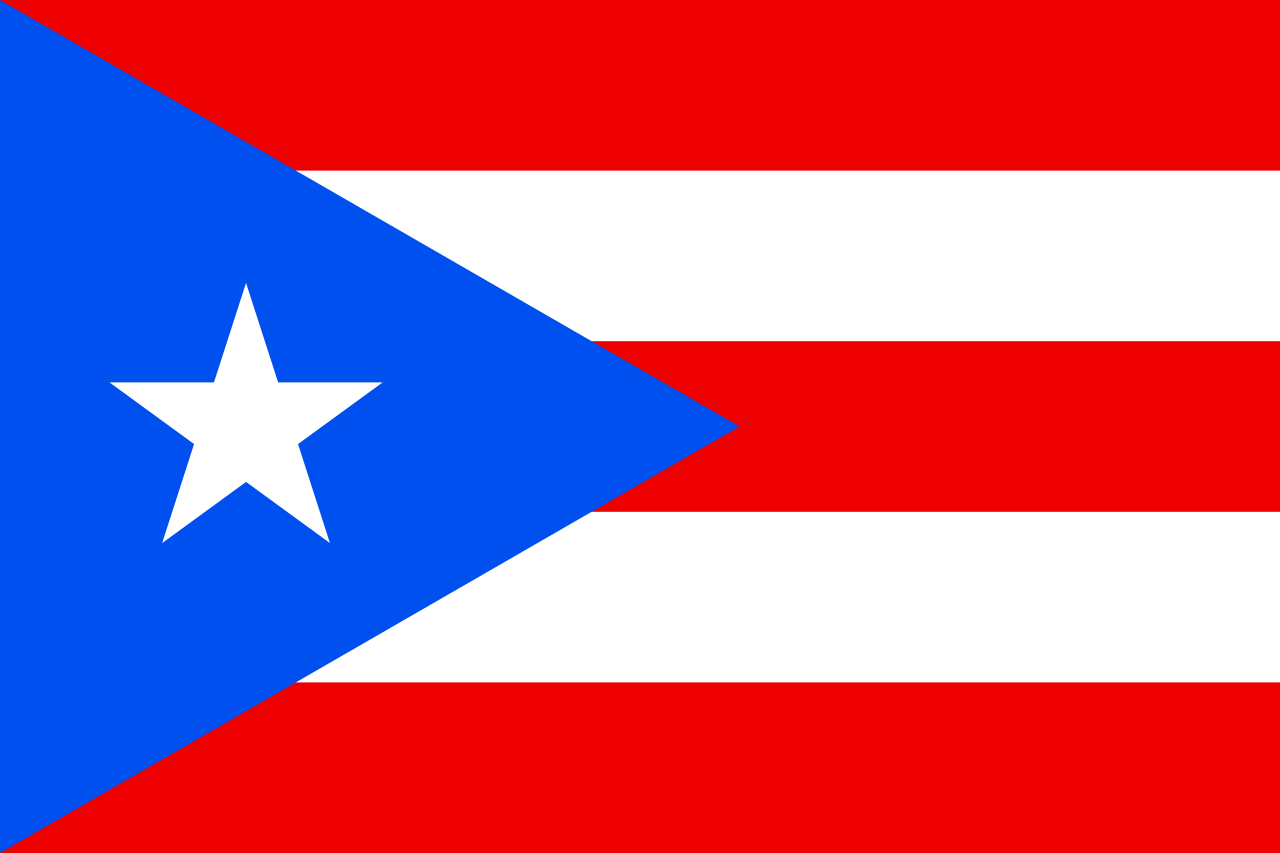西班牙语字母 A a (Á á) B b C c (Ch ch) D d E e (É é) F f G g H h I i (Í í) J j K k L l (Ll ll) M m N n Ñ ñ O o (Ó ó) P p Q q R r S s T t U u (Ú ú) (Ü ü) V v W w X x Y y Z z